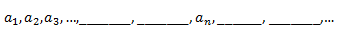## Wednesday, April 25, 2012

### We're getting closer with recursive definitions

Last semester was the first time I used a College Algebra curriculum that taught sequences and series.  The first section of this chapter was a nice little intro to sequences and series, just to get students used to notation.  I thought I did such a good job explaining sequences that are defined recursively.  Until I saw the test.

Not.  So.  Hot.

Then, as I helped students in our Math Lab, I realized something--recursive definitions are not that obvious to students.

I think what happened here was a classic case of it's-so-obvious-to-the-teacher-she-automatically-thinks-it's-obvious-to-everyone-else.  We've all had teachers like this.  My absolute favorite prof from grad school loved the phrase, "Oh, this is kindergarten stuff!"  Which usually had one of two effects on me:  (1) Ahhhh!!  This is NOT kindergarten stuff!  I just spent the majority of my weekend trying to figure this out!  (2)  Where in the world did you go to kindergarten?  Remind me to send my kids there.

But I digress.

What hit me was that when I see something like:
an=an1+an2

I automatically think, "If I want to find a certain term, I need to sum up the two previous terms."  Furthermore, I know that
an+1=an+an1

means the same thing as the previous equation.

On the other hand, when my students saw a recursive definition, I'm pretty sure they thought, "WTF.  Skip it."

So, this semester I paid much more attention to these types of sequences.  The very first thing I did regarding recursive definitions was show a slide with this at the top:I asked students to fill in the blanks and then asked them three questions:
1. What do the dot, dot, dots mean?
2. What do we call the term before a_n?
3. What about the term after a_n?
Maybe this is an obvious starting point, but it was such a revelation to me.

We then did some examples with

an=an1+an2
I had them tell me what they thought it meant (with a lot of guidance from questions like, "a_(n-1) is related to a_n how?").  Then we wrote a few equations in symbols and in words.  For example for,

a4=a3+a2

a
n
=an1+an2

I made them write "The fourth term is equal to the third term plus the second term."  And so on.

Then came
an+1=an+an1

which everyone was convinced was a totally new problem (darn you, indices!).  But, once we did the same examples (finding a_4, etc.), I think/hope all minds were changed.

After working some specific examples, where initial values were given, I gave them an exit ticket of something like:

List the first five terms of the sequence defined by:
a_1 is the number of boys in the room; a_2 is the number of girls;

an=an1+2an2
Finj
I think about 80% of students got it with zero help from me.  Not perfect, but I'll take it this time around!

1.2.